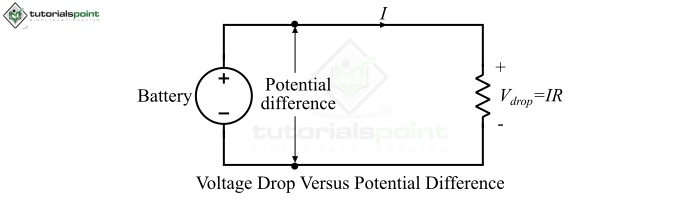# Difference between Voltage Drop and Potential Difference

While analyzing electric circuits, we come across the electrical quantities "voltage drop" and "potential difference" that appear to be the same at first sight because both are measured in Volts. In this article, we will highlight how Voltage Drop is different from Potential Difference in many aspects.Before discussing the differences between Voltage Drop and Potential Difference, let's start with some basics so that it becomes easy to understand the differences between them.

## What is Voltage Drop?

When an electric current flows through a circuit and there is a drop or decrease in the electric potential of the charge carriers along the path of the current, it is known as voltage drop or potential drop.

Difference between Voltage Drop and Potential Difference

The resistor is the main electric circuit component which causes the voltage drop when an electric current passes through it. If R is the resistance of a resistor and I is the current flowing through it, then the voltage drop across the resistor is given by,

$$V\:=\:IR$$

The voltage drop is measured in Volts. The voltage drop is the function of resistance of the circuit element, therefore, the voltage across different element is different. The voltage drop of any electric circuit element or a resistor can be measured by using a voltmeter.

## What is Potential Difference?

In an electric circuit, the difference in the potential of two points is known as potential difference (P.D.). The potential difference is also known as voltage. The SI unit potential difference is Volt.

In simple terms, the potential difference can be defined as the arithmetical difference of a higher potential and a lower potential in an electric circuit. Basically, the potential difference is the amount of energy required to move a unit charge from one point to another point in an electric circuit. The potential difference between two points in an electric circuit can be established with the help of a source of EMF like cell, battery, etc. Just like the voltage drop, we can use a voltmeter to measure the potential difference in the circuit.

Mathematically, if we require W joules of energy (or work done) to move an electric charge of Q coulombs from one point to another in an electric circuit, then the potential difference between those two points is given by,

$$V\:=\:\frac{W}{Q}$$

Potential Difference is the parameter in any electric circuit which is entirely responsible for the flow of current in the circuit. Thus, if there is no potential difference, then no current flow.

## Difference between Voltage Drop and Potential Difference

Both these quantities "voltage drop" and "potential difference" are denoted by the same symbol "V" and are measured in the same unit, i.e. Volts. However, there are several differences between voltage drop and potential difference that are highlighted in the following table:

Basis of Difference Voltage Drop Potential Difference
Definition The decrease in the electric potential of charge carriers along the path of electric current in an electric circuit is known as voltage drop. The difference of electric potential between two points in an electric circuit is known as potential difference. It is also known as voltage.
Responsible circuit elements Voltage drop occurs due to impeding circuit elements (that oppose the current flow) like resistor, inductor, capacitor, etc. Potential difference occurs due to elements that are the source of emf like cell, battery, etc.
Part of occurrence Voltage drop occurs across a particular circuit element. Potential difference occurs between any two points in an electric circuit.
Main cause of existence The main cause of voltage drop is the current flowing through a circuit element. The main cause of potential difference is EMF (Electromotive Force).
Formula

Voltage drop is given by

$$V\:=\:IR$$

Where, I is the current and R is the resistance of path of current flow.

Potential difference is given by, $$V\:=\:\frac{W}{Q}$$

Where, W is the energy or work done to move charge and Q is the charge.

Physical meaning Voltage drop is a decrease in the energy of charge carriers due to electric friction Potential difference is the energy expended by a charge carrier to travel from one point to another.
Dependency on current Voltage drop can occur only when there is a flow of electric current through the element. Potential difference can exist even without the flow of current in the circuit.
Considered as Voltage drop is considered as a loss due to resistance or impedance of the circuit. Potential difference is considered as the difference of potential energy of two different points in a circuit.

## Conclusion

To conclude, Voltage Drop is the decrease in the potential energy of change carriers along the path of current, whereas Potential Difference is the amount of energy required by a charge carrier to travel from one point to another in a circuit. Both "voltage drop" and "potential difference" are denoted by the same symbol "V" and are measured in the same unit, i.e., Volts.